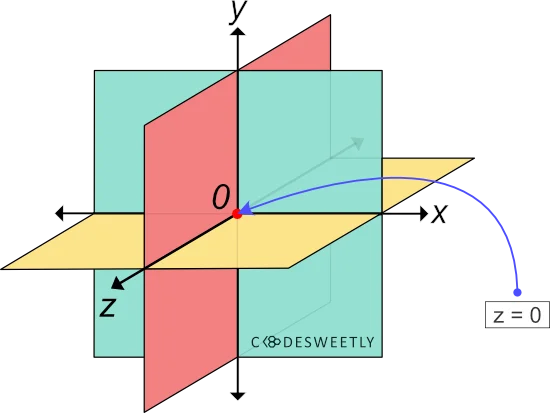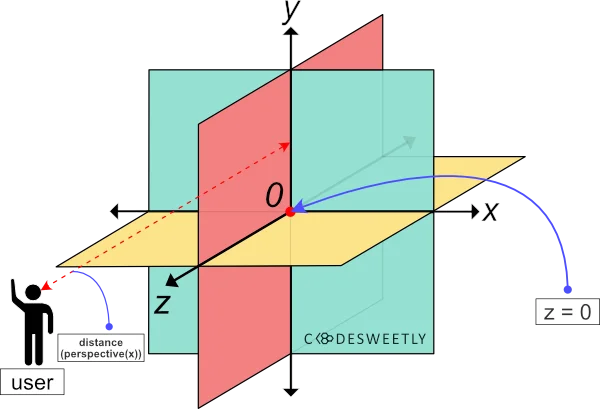# CSS perspective() Function – How to Add Perspectives to Elements

perspective() transforms an element by adding some perspective effects to it.

## Syntax of the CSS perspective() Function​

perspective() accepts only one argument. Here is the syntax:

element {  transform: perspective(length);}

The length argument specifies the user's distance to the z=0 plane.

## What Is the z=0 Plane?​

The z=0 plane is the point on the Cartesian coordinate system where the coordinate z equals zero (0).A three dimensional Cartesian coordinate system with an arrow pointing to the z=0 plane

## z=0 Plane vs. perspective(): What Is the Difference?​

The z=0 plane is the point on the z-axis where z equals zero (0).

The CSS perspective() function defines the distance between the user and the imagery plane located at the coordinate z=0.

In other words, suppose you pass a positive length argument to the CSS perspective() function. In that case, the greater the length, the farther the distance between you and the z=0 plane.

For instance, a perspective(70px) method creates a 70px distance between you and the z=0 plane. While a perspective(300px) method creates a 300px distance.A three dimensional Cartesian coordinate system with a red arrow defining the distance between the user and the z=0 plane
info
• The perspective() method's argument must be a positive value. Otherwise, the browser will apply no perspective effect on the selected element.
• List perspective() first whenever you chain it with other CSS transform functions. Otherwise, browsers might transform the selected element incorrectly.

## Examples of the CSS perspective() Function​

We often use perspective() with other CSS functions such as translateZ(), rotateX(), and rotateY(). Below are some examples.

### How to use perspective() with the CSS translateZ() function​

img {  width: 40%;}.second-image {  transform: perspective(33px) translateZ(10px);}

Try Editing It

Here's what we did in the snippet above:

1. We used the perspective() function to define a 33px distance between the user and the z=0 plane.
2. We used the translateZ() function to reposition the second-image ten pixels (10px) away from its original position along the z-axis.

Note the following:

• Suppose the second-image's z-axis position is larger than or equal to the perspective() function's argument. In that case, the image will disappear as though it is behind the user. In other words, the selected item disappears when the user is in the same position as the element. Or when the element is behind the user.
• The larger the user's distance to the element's z-axis position, the less intensive the perspective effect will be, and vice-versa.

### How to use perspective() with the CSS rotateY() function​

img {  width: 40%;}.second-image {  transform: perspective(33px) rotateY(-10deg);}

Try Editing It

Here's what we did in the snippet above:

1. We used the perspective() function to define a 33px distance between the user and the z=0 plane.
2. We used the rotateY() function to rotate the second-image negative ten-degree (-10⁰) around the y-axis.

### How to use perspective() with the CSS rotateX() function​

img {  width: 40%;}.second-image {  transform: perspective(33px) rotateX(17deg);}

Try Editing It

Here's what we did in the snippet above:

1. We used the perspective() function to define a 33px distance between the user and the z=0 plane.
2. We used the rotateX() function to rotate the second-image seventeen-degree (17⁰) around the x-axis.

## CSS perspective() Function vs. perspective Property: What's the Difference?​

The CSS perspective() function and the perspective property provide two similar ways to add perspective effects to HTML elements.

The main differences between the two perspective techniques are as follows:

• We apply the perspective() function "directly on the element" we want to add some perspective effects to.
• We apply the perspective property "on the parent element" of the element we want to add some perspective effects to.
• The perspective() function works as a transform property's value.
• The CSS perspective property allows you to create perspective effects without using the CSS transform property.

Here's an example:

Use CSS perspective property to add perspective effect to a child element:

img {  width: 40%;}div {  perspective: 33px;}.second-image {  rotate: x 17deg;}

Try Editing It

Here's what we did in the snippet above:

1. We used the perspective property to define a 33px distance between the user and the z=0 plane.
2. We used the rotate property to rotate the second-image seventeen-degree (17⁰) around the x-axis.
note
• The CSS perspective property saves you from remembering the specific order to position the transform functions.
• A none value tells browsers not to add any perspective effect to the selected element's children.

## Overview​

This article discussed what a CSS perspective() function is. We also used examples to see how it works.